## Friday, 11 December 2009

### If Zero by Zero were defined....

If zero by zero were defined a whole lot of crazy things would start to happen.
Just as an example I quote this section of Wikipedia.

With the following assumptions: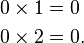\begin{align} 0\times 1 &= 0 \\ 0\times 2 &= 0. \end{align}
The following must be true: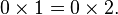$0\times 1 = 0\times 2.\,$
Dividing by zero gives: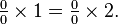$\textstyle \frac{0}{0}\times 1 = \frac{0}{0}\times 2.$
Simplified, yields: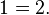$1 = 2.\,$
The fallacy is the implicit assumption that dividing by 0 is a legitimate operation with 0/0 = 1.
Although most people would probably recognize the above "proof" as fallacious, the same argument can be presented in a way that makes it harder to spot the error. For example, consider the following equations: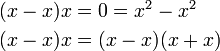\begin{align} (x-x)x &= 0 = x^2-x^2 \\ (x-x)x &= (x-x)(x+x) \end{align}
Dividing by x − x gives: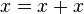$x = x+x\,$
and dividing by x gives:$1 = 2.\,$
Therefore it would  be possible to prove that 1 = 2 and 2=3. In fact it would be within our capability to prove that any number in the number system is equal to any other number. If this were true then everything would be exactly the the same.It is therefore obvious that division by zero is undefined. If zero by zero were defined all the buildings and bridges would tumble and crumble. All calculations would be rendered useless. You wouldn't be able to tell the difference between an elephant and an ant. If zero by zero were defined, there would be no difference between you and me, no difference between big and small, no difference between hot and cold. The universe would be astoundingly uniform. There would be no stars or galaxies or beautiful nebulae. No black holes, no Quasars, no interstellar dust. The universe would be just one big boring entity.

Evee said...

VERY. VERY. VERY. interesting post.

I guess it just brings it all down to...

admitting that math has errors too, as well. :P

Srikanth said...

like we proved before 0/0 is mathematical everything. If it can be defined its values would include nothing, every other value and infinity!

Ashwin said...

-Evelyn
It's not that math has errors. If it did, all our calculations would be useless and all our buildings would start collapsing. Zero by zero is undefined because it is not logical to extract nothing out of nothing. It is similar to this situation: Imagine that I have an argument with which I can prove that an apple and a banana are the same thing. It follows logically that my argument must be wrong because it can be verified by experiment that an apple is not a banana. The fact that 0/0 is undefined just proves that you have to think about whether a mathematical operation is logical just like you have to think about whether a statement about your character is logical before you accept it.

-Srikanth

Yes this goes back to the good old days when we theoretically proved that Nothing in itself is Something and therefore Something must be equal to Nothing. And since Something is Everything
Nothing, Something and Everything are exactly the same and in this case it is 0/0. But of course this is true only if zero / zero were defined and it isn't. But we liked to think that it is.